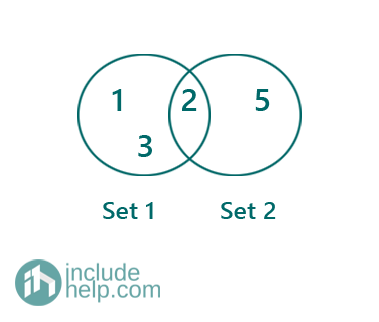Submitted by Radib Kar, on September 11, 2020

Disjoint set is an important mathematical concept which is an abstract data structure too. Disjoint sets mean a collection of elements without any specific order. To implement disjoint set ADT, an array is enough also. ADT set is an auxiliary data structure used to solve many algorithmic problems based on graphs.

The basic operations on a disjoint set are the below ones:

1. MAKESET(X): create a new set with element X
2. UNION(X, Y): creating a set with X & Y and it deletes individual sets of X & Y.
3. FIND(X): It finds the set in which X is there. (X can't be in two different sets since it's named as disjoint set ADT)The above is an example of disjoint set ADT whereas the below one is not since it's not disjoint.For the below disjoint sets we show the ADT operations,

1. MAKESET(X), MAKESET(Y) creates two disjoint sets having element X & Y.
Like below:2. FIND(X): It returns name of the set where X exists which is here set1
3. UNION(X, Y): It creates a new set with element X & Y and removes the old sets of X & Y. so UNION(X, Y) returns the below:## Application of disjoint set ADT

1. Representing network connectivity
2. Finding cycle in an undirected graph
3. Kruskal's Minimum spanning tree algorithm
4. In gaming algorithms'

Here we saw the theoretical concepts behind the disjoint set ADT and in further articles we are going to application of disjoint set ADT.

Disjoint Set problems (programs on Disjoint Set)

Languages: » C » C++ » C++ STL » Java » Data Structure » C#.Net » Android » Kotlin » SQL
Web Technologies: » PHP » Python » JavaScript » CSS » Ajax » Node.js » Web programming/HTML
Solved programs: » C » C++ » DS » Java » C#
Aptitude que. & ans.: » C » C++ » Java » DBMS
Interview que. & ans.: » C » Embedded C » Java » SEO » HR
CS Subjects: » CS Basics » O.S. » Networks » DBMS » Embedded Systems » Cloud Computing
» Machine learning » CS Organizations » Linux » DOS
More: » Articles » Puzzles » News/Updates